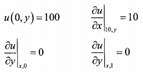Create an Account

Home / Questions / 1 Solve Laplace& 39 s equation with the following boundary conditions and discuss th...

1 Solve Laplace& 39 s equation with the following boundary conditions and discuss the results 2 Develop the finite difference approximation of

1.       Solve Laplace&#39;s equation with the following boundary conditions and discuss the results:2. Develop the finite difference approximation of Fick&#39;s second law of diffusion in polar coordinates. Write a MATLAB program that can be used to solve the following problem: A wet cylinder of agar gel at 278 K with a uniform concentration of urea of 100 mol/m3 has a diameter of 0.03 m and is 1.0 m long with flat parallel ends. The diffusivity is 5×10 -1 m2/s. Calculate the concentration at the midpoint of the cylinder after 100 h for the following cases if the cylinder is suddenly immersed in turbulent pure water. Since the cylinder is long, you may ignore axial diffusion.

Jul 23 2020 View more View LessSubscribe To Get Solution